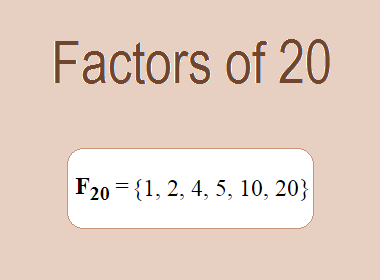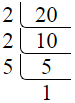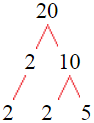# Factors of 20The factors of 20 are 1, 2, 4, 5, 10, and 20 i.e. F20 = {1, 2, 4, 5, 10, 20}. The factors of 20 are all the numbers that can divide 20 without leaving a remainder.

We can check if these numbers are factors of 20 by dividing 20 by each of them. If the result is a whole number, then the number is a factor of 20. Let's do this for each of the numbers listed above:

·        1 is a factor of 20 because 20 divided by 1 is 20.

·        2 is a factor of 20 because 20 divided by 2 is 10.

·        4 is a factor of 20 because 20 divided by 4 is 5.

·        5 is a factor of 20 because 20 divided by 5 is 4.

·        10 is a factor of 20 because 20 divided by 10 is 2.

·        20 is a factor of 20 because 20 divided by 20 is 1.

## How to Find Factors of 20?

1 and the number itself are the factors of every number. So, 1 and 20 are two factors of 20. To find the other factors of 20, we can start by dividing 20 by the numbers between 1 and 20. If we divide 20 by 2, we get a remainder of 0. Therefore, 2 is a factor of 20. If we divide 20 by 3, we get a remainder of 2. Therefore, 3 is not a factor of 20.

Next, we can check if 4 is a factor of 20. If we divide 20 by 4, we get a remainder of 0. Therefore, 4 is a factor of 20. We can continue this process for all the possible factors of 20.

Through this process, we can find that the factors of 20 are 1, 2, 4, 5, 10, and 20. These are the only numbers that can divide 20 without leaving a remainder.

********************

********************

## Properties of the Factors of 20

The factors of 20 have some interesting properties. One of the properties is that the sum of the factors of 20 is equal to 42. We can see this by adding all the factors of 20 together:

1 + 2 + 4 + 5 + 10 + 20 = 42

Another property of the factors of 20 is that the prime factors of 20 are 2, and 5 only.

## Applications of the Factors of 20

The factors of 20 have several applications in mathematics. One of the applications is in finding the highest common factor (HCF) of two or more numbers. The HCF is the largest factor that two or more numbers have in common. For example, to find the HCF of 20 and 24, we need to find the factors of both numbers and identify the largest factor they have in common. The factors of 20 are 1, 2, 4, 5, 10, and 20. The factors of 24 are 1, 2, 3, 4, 6, 8, 12, and 24. The largest factor that they have in common is 4. Therefore, the HCF of 20 and 24 is 4.

Another application of the factors of 20 is in prime factorization. Prime factorization is the process of expressing a number as the product of its prime factors. The prime factors of 20 are 2, and 5 since these are the only prime numbers that can divide 20 without leaving a remainder. Therefore, we can express 20 as:

20 = 2 × 2 × 5

We can do prime factorization by division and factor tree method also. Here is the prime factorization of 20 by division method,20 = 2 × 2 × 5

Here is the prime factorization of 20 by the factor tree method,20 = 2 × 2 × 5

## Conclusion

The factors of 20 are the numbers that can divide 20 without leaving a remainder. The factors of 20 are 1, 2, 4, 5, 10, and 20. The factors of 20 have some interesting properties, such as having a sum of 42. The factors of 20 have several applications in mathematics, such as finding the highest common factor and prime factorization.